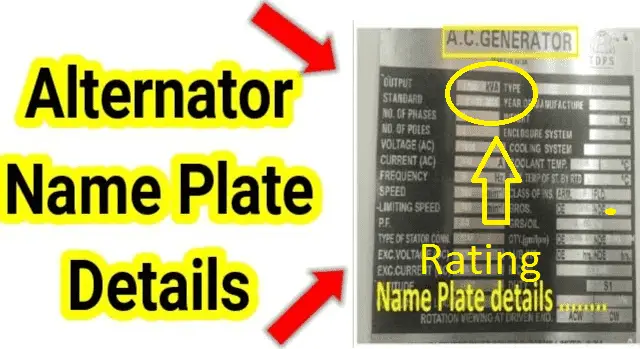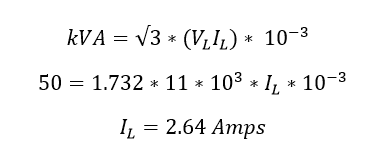# Why Are Alternators Rating in KVA or MVA

## Why are alternators rating in KVA?### Reason 1

The alternator is an energy conversion device, which converts mechanical energy into electrical energy. It is working under Faraday’s law of electromagnetic induction. Here the alternators are designed to supply a specific voltage for various loads. The output voltage of an alternator is called VL volts. The power drawn in the alternator depends on its power factor, in alternator you can vary the power factor by adjusting the field input current and voltage

When you lower the field voltage (AVR output) the power factor increases

At the same time when you rise the field voltage the power factor decreases

Hence instead of specifying rating of alternator in watts, it is specified in terms of the maximum apparent power which it can supply to the load. In three phase circuits, the apparent power is root 3*VL IL Here (IL is the output current of an alternator) the apparent power is measured in Volt Amps (VI). This is generally expressed in kilo volt amperes and is called kVA rating of an alternator where lL , is the rated full load current supply. So for a given rated voltage and kVA rating of an alternator, its full la rated current can be decided.

### Reason 2:

The reason for which the transformers and alternator (synchronous generators) are rated in volt-amperes instead of watts is that manufacturer does not know at what power factor does these equipments are going to operate. On the other hand, while manufacturing the motors manufacturer specifies the power factor at which the motor should be operated. Most of the power manufacture takes 0.8 to 0.86 as a default power factor. This is the reason why the motor loads (including synchronous motors are rated in kw) are rated in wattage power.

In the case of alternator and transformers manufacturer does not know the operating power factor. Hence they are rated in KVA or MVA

Consider a generator whose output power is 1000kVA, for different power factors 0.2, 0.5 and 0.8 and unity respectively the output power in kW will be 200, 500, 800 and 1000kW. The power factor can be vary by adjusting the output of the AVR (automatic voltage controller)